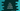# C++ program to create a simple calculator program## C++ program to create a simple calculator program:

In this program, we will write one simple calculator program in C++. This program can do addition, subtraction, division and multiplication with two numbers. The user will enter the numbers and mode via terminal.

With this program, you will learn how to take user inputs, how to use a switch block and how to do arithmetic operations in C++.

### C++ program:

Below is the complete C++ program:

``````#include <iostream>

using namespace std;

int main()
{
int firstNumber, secondNumber;
int mode;

cout << "Enter 1 for addition, 2 for subtraction, 3 for multiplication and 4 for division :" << endl;
cin >> mode;

cout << "Enter the value of first number and second number :" << endl;
cin >> firstNumber >> secondNumber;

switch (mode)
{
case 1:
cout << firstNumber << " + " << secondNumber << " = " << firstNumber + secondNumber << endl;
break;
case 2:
cout << firstNumber << " - " << secondNumber << " = " << firstNumber - secondNumber << endl;
break;
case 3:
cout << firstNumber << " * " << secondNumber << " = " << firstNumber * secondNumber << endl;
break;
case 4:
cout << firstNumber << " / " << secondNumber << " = " << firstNumber / secondNumber << endl;
break;
default:
cout << "Invalid input";
break;
}
}``````

### Explanation:

In this program,

• firstNumber and secondNumber variables are used to store the first and the second number.
• mode is used to store the calculator mode, i.e. addition, subtraction etc.
• It asks the program to enter the mode at first and stores that value in mode variable.
• Then, it asks the user to enter the first and the second number. These numbers are stored in firstNumber and secondNumber variables.
• Finally, it enters a switch-case block. Based on the value of mode, it moves to the respective case block and does the operation. For any invalid inputs, it prints one message.

### Sample output:

It gives output as like below:

``````Enter 1 for addition, 2 for subtraction, 3 for multiplication and 4 for division :
2
Enter the value of first number and second number :
40 20
40 - 20 = 20``````